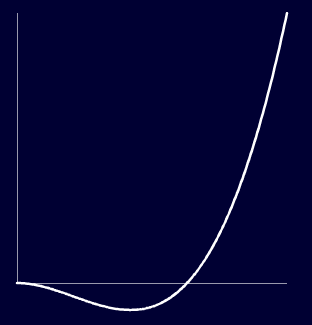用Itween很久了，但是Itween有时候会有动画执行到一半卡住的问题，于是就想自己写一个动画系统来取代itween，但是又希望新的动画系统也能像itween上有许多丰富的插值算法，于是找了一些资料，把研究结果记录于下。

首先是itween算法的官方文档
itween 插值算法 官方文档 Lua(版)

关于itween插值算法的效果可以去这个网站查看
itween 插值算法 演示

这里分析几个itween的插值算法

``````//平方淡入
local function inQuad(t, b, c, d)
t = t / d
return c * pow(t, 2) + b
end
``````

t = Time (经过的时间)
b = Begin (开始值)
c = Change (变化值)
d = Duration （动画总时间）

t = 0 时
`return c * pow(0,2) + b`
`= c * 0 + b`
`= b`

t = 1 时
`return c * pow(1,2) + b`
`= c * 1 + b`
`= c + b`

``````//回弹淡入
local function inBack(t, b, c, d, s)
if not s then s = 1.70158 end
t = t / d
return c * t * t * ((s + 1) * t - s) + b
end
``````

t= 0 时
`return c * 0 * 0 * ((s + 1) * 0 - s) + b`
`= b`

t = 1 时
`return c * 1 * 1 * ((s + 1) * 1 - s) + b`
`= c * ( s - s + 1) + b`
`= c + b`

`c * pow(t , 2) * ( (s + 1) * t - s)`

t = s /(s + 1) 时，函数值为0
t = s /(s + 1) 时，函数值为正

s = 1.70158 时 s /(s + 1) 约等于0.62基本上符合我们的预期，并且我们可以调整s的值来使我们回弹动画更快或者更慢。

ok,我这篇文章就到这里，更多的插值效果请看官方文档 ^_^